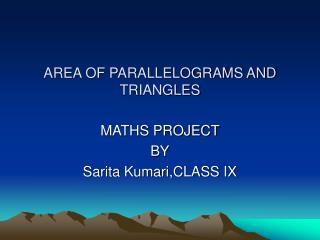DownloadDownload PresentationAREA OF PARALLELOGRAMS AND TRIANGLES

# AREA OF PARALLELOGRAMS AND TRIANGLES

Télécharger la présentation## AREA OF PARALLELOGRAMS AND TRIANGLES

- - - - - - - - - - - - - - - - - - - - - - - - - - - E N D - - - - - - - - - - - - - - - - - - - - - - - - - - -
##### Presentation Transcript

1. AREA OF PARALLELOGRAMS AND TRIANGLES MATHS PROJECT BY Sarita Kumari,CLASS IX

2. PLANAR REGION AND CONGRUENT FIGURES • PLANAR REGION- The part of the plane enclosed by simple closed figure is called a planar region. • CONGRUENT FIGURES- Two figures are called congruent, if they have the same shape and the same size.

3. PARALLELOGRAMS • 1.Parallelograms on the same base and the between the same parallels are equal in area. • 2.Adiagonal of a parallelogram divides it into two triangles of equal area. • 3.Parallelograms and a rectangle on the same base and between the same parallels are equal in area.

4. PARALLELOGRAMS • 4.The area of a parallelogram is the product of its base and the corresponding altitude. • 5.The area of a triangle is half he product of any of its side and the corresponding altitude. • 6.If a triangle and a parallelogram are on the same base and between he same parallels,the area of the triangle is equal to half of he parallelogram.

5. TRIANGLES • 1.Triangles on the same base and between the same parallels are equal in area. • 2.Triangles having equal areas and having one side of one of the triangles equal to one side of the other have their corresponding altitudes equal. • 3.Two triangles having the same base and equal areas lie between the same parallels.

6. THANK YOU